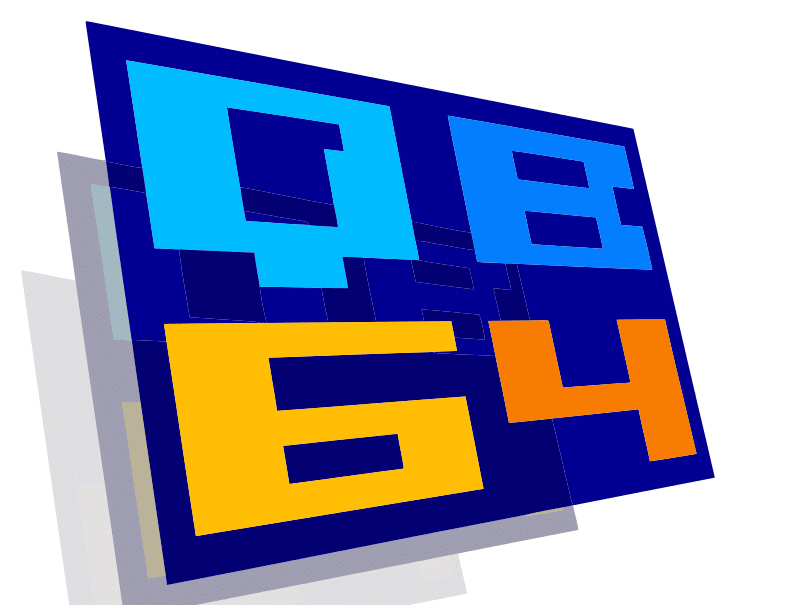# Learning QBasic: Calculator

No account yet? Register

Do you want to learn QBasic? Below is an example of it. The program below accepts two numbers and performs a math calculation on it, similar to a calculator.

QBasic stands for Quick Beginners All Purpose Symbolic Instruction Code. It was created by Microsoft in 1991 and is based on the original BASIC language released by them in 1981. BASIC was often the first language home programmers learned and used. QBasic is a great language for beginners to start learning how to code. You only need a Windows computer to run it and just need to install the software. If you have a Mac computer you can use an emulator, like DOSBox to run it.

```CLS

PRINT “ANY NAME YOU WANT”

INPUT “Enter two numbers.”; nm, nm2

PRINT “Choose OPERATION:”

PRINT “1. Add 2. Subtract 3. Multiply 4. Divide “

INPUT operation

IF operation = 1 THEN PRINT “The result is”; nm + nm2

IF operation = 2 THEN PRINT “The result is”; nm– nm2

IF operation = 3 THEN PRINT “The result is”; nm * nm2

IF operation = 4 THEN PRINT “The result is”; nm / nm2
CLS
PRINT " THANKS FOR USING MY PROGRAM"

END ```

I think it is great.

In order to run it, you need to install QBasic on your computer. Here is a video explaining how to do it. Were you able to get my code to run?